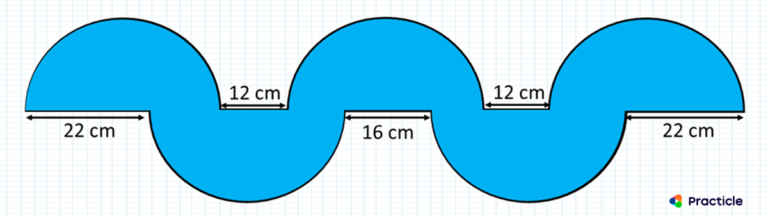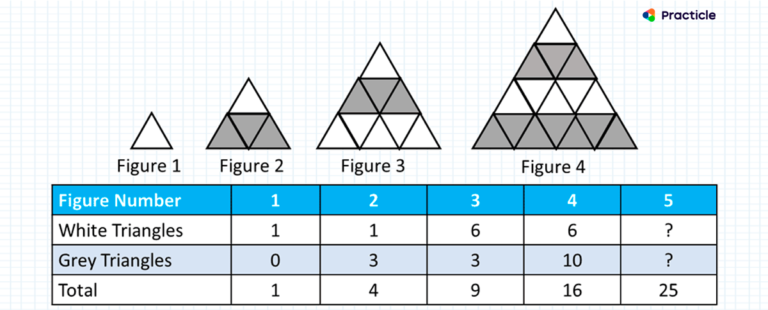# 2019 PSLE Maths Questions & Answers

The 2019 PSLE Maths Questions has caused quite a stir amongst Primary 6 parents and students.

You might have heard people discussing the answers to the semicircle question, the egg tart question and the triangle question. These questions have been spreading like wild fire ever since there were news of them “leaving pupils in tears” (Source: The Straits Times).

If you are wondering how difficult these 2019 PSLE Math questions really are and are game for a challenge, here are the questions.

### 1. 2019 PSLE math question on semi circlesThe figure consists of 5 identical semicircles arranged as shown. Find the diameter of one of the semicircles.

*Note that this might not be the actual phrasing of the question in PSLE.

This Math question is under the topic of Circles in the Primary 6 Math syllabus. It provides the students with a set of semicircles and measurements, and our Primary 6 students need to find the diameter of a semicircle.

### How to solve this PSLE 2019 semi circle question

The key to answering this question lies in thinking critically and using some spatial visualization skills.

Think about how the diameters of the 5 semi circles are linked to one another.

#### How this semicircle question can be difficult to Primary 6 children:

A reason that some students get stuck on this particular semicircle PSLE question is because the distance between the semi circles is slightly different and they might not have encountered such a scenario before.

### 2. 2019 PSLE math question on Patterns

a. Fill in the table above
b. Find the total number of grey and white triangles that Figure 250 has.
c. What is the percentage of grey triangles in Figure 250?*Note that this might not be the actual phrasing of the question in PSLE.

### How to solve this PSLE 2019 pattern problem

The trick to answering this PSLE triangle question is to really look at the pattern and understand how the information that is given relate to one another.

To figure out the correct answer for this question, we can go back to the fundamentals of model drawing.

#### Why such a pattern question may be difficult to Primary 6 children:

The P6 students are presented with a few figures that follow a certain pattern in this question, and there are 3 parts involved. Most of the Primary 6 students are able to solve the first 2 parts, but get stuck at part c of the question.

Some students are unable to solve the last part of this PSLE triangle question because they are used to doing Math pattern questions with a consistent difference. However, this question presents a unique situation whereby the number of white and grey triangles alternate.

### 3. 2019 PSLE math egg tart question

Jamie and Oliver used \$61.20 each to buy some egg tarts. Jamie has a 15% discount coupon and bought 6 more egg tarts than Oliver.
a. How many egg tarts did Jamie buy?
b. What is the cost of an egg tar without the discount?

This egg tart question provides the students with a situation where 2 children bought some egg tarts and our Primary 6 students need to apply what they have learnt about percentages to solve the problem.

*Note that this might not be the actual phrasing of the question in PSLE.

### How to solve this PSLE 2019 percentage problem

#### Why this egg tart question may be difficult to Primary 6 children:

This egg tart question requires a clear understanding on how percentages are applied to real life.

To answer it, you need to think of the cost of the egg tarts in terms of the percentage of the price one of them paid.

### Conclusion

How many of these questions did you get correct? Were they hard or “tricky” to you?

Although these 3 questions are not the typical straightforward word problem sums, they do encourage our Primary 6 children to think more creatively and apply their Math problem-solving skills in specific situations, just like our Math questions in Practicle.

If you want to help your child develop the right attitude to problem solve and learn to think about problems from different angles, check us out today.